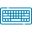# Inverse Laplace Transform Calculator## Table of Content

Feedback

The inverse Laplace transform calculator is a digital tool that works backward to reveal the original mathematical expressions (real variables) from a transformed version called Laplace Transform. This tool aids in understanding the behavior of systems described in the Laplace domain.

## Concepts of Inverse Laplace Transform:

The inverse Laplace transform is a method in which we can revert back from the frequency domain F(s) to the time domain f(t).

Inverse Laplace Transforms are, as its name suggests, the inverse operation of Laplace Transforms.

The Laplace Transform helps you change a function from a time domain f(t) to a frequency domain F(s). On the other hand, the inverse Laplace Transform reverses this transformation.

Inverse Laplace transform is also identified as inverse Laplace transformation or inverse Laplace and expressed as $$L^{-1}f(t)$$.

## Inverse Laplace Transform Expressions:

Here's the formula to calculate the inverse Laplace transform,

$$f(t) = \mathcal{L}^{-1}F(s)$$

In this formula:

$${f}{(t)}$$ represents the original function in the time domain.

$$L^{-1}$$ denotes the inverse Laplace transform.

$${F}{(s)}$$ stands for the transformed function in the Laplace domain.

## Laplace Inverse Table:

Here's a table of common examples of inverse Laplace transforms,

Laplace Transform (F(s)) Inverse Laplace Transform (f(t))
1 δ(t) (Dirac delta function)
e^(at) 1/(s-a)
t^n (for n ≥ 0) n!/(s^(n+1))
e^(at)sin(bt) b/((s-a)^2 + b^2)
e^(at)cos(bt) (s-a)/((s-a)^2 + b^2)
1/(s-a) e^(at)
1/(s^2) t
1/(s(s-a)) 1 - e^(at)

## Inverse Laplace Transform Calculations & Examples:

We are going to solve an example through which you can easily calculate the inverse Laplace transform.

### Example :

Let's find the inverse Laplace transform:

$$F(s) = \frac{12}{s} - \frac{1}{s - 10} + 8(s - 25)$$

Upon examination of the terms, we notice that the denominator of the first term is simply a constant. The correct numerator for this term is "1". When using the inverse Laplace Transform Calculator with steps, we will only consider the factor 12 before performing the inverse transformation. Therefore, a = 10 is the correct numerator, just as it needs to be.

The third term appears to be exponential, but this time,  a = 25. Before performing the inverse transformation, we need to factor in the 8.

In more detailed form:

$$F(s) = \frac{12}{s} - \frac{1}{s - 10} + 8(s - 25) f(t) = 12(1) - e^{10t} + 8e^{25t} = 12 - e^{10t} + 8e^{25t}$$

So, the inverse transform of $${F}{(s)} is {f}{(t)} = 12 - e^{10t} + 8e^{25t}$$. You can also try the inverse Laplace calculator for hassle-free and faster results.

## Steps to Use Inverse Transform Calculator:

If you don’t want to solve manually then you can use this free online inverse laplace transform calculator for this. All you need to do is follow simple steps which include:

What to do:

• Enter a complex function F(s) and see the equation preview in Laplace form.
• Press the Calculate button.

What you get:

• The inversed version of the given Laplace equation.

## FAQs:

### What are the properties of inverse Laplace?

Here are some properties that describe the inverse Laplace of a function.

• Linearity Property.
• Change of Scale Property.
• Shifting Property
• Second Shifting Property
• Property of Inverse Laplace Transform of Integrals.
• Property of Inverse Laplace Transform of Derivatives.
• Property of Multiplication by the Powers of s.

### What are the different types of Laplace transforms?

There are two types of Laplace transformation such as,

• One-sided Laplace transformation
• Two-sided Laplace transformation

## References:

Libretexts.org: The Inverse Laplace Transform and Definition of the Inverse Laplace Transform### Alan Walker

Studies mathematics sciences, and Technology. Tech geek and a content writer. Wikipedia addict who wants to know everything. Loves traveling, nature, reading. Math and Technology have done their part, and now it's the time for us to get benefits.